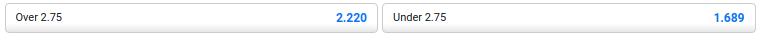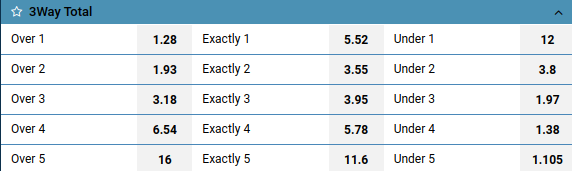# Total Under Over odds in sports betting

Total is bets on the final total number of indicators of a sporting event. For example, in football, this is often the total of goals, in basketball - points, and in tennis - sets. For the sake of convenience, further examples, we will most often consider the totals for goals in a football match.

## Types

Total is one of the most popular bets in the odds line of any bookmaker. Often the size of the line per total is very varied, so below we will try to describe the main types.

### Total Over and Total Under

In this type of total, the final total number of indicators must be less / more than the specified value of the additional value.

Let's say we bet on “Under 1.5” goals in football. Now let's consider the possible final score of the match and the results.• The score in the match is 0-0: we will win because the total of goals is 0, which is less than 1.5.
• 1-0: we win, since the total of goals is 1, which is also less than 1.5.
• 1-1: we lose, since the total of goals is 2, which is more than 1.5.
• 2-0, 3-1: we lose, since the total number of goals is more than 1.5.
For “Total Over 1.5” everything works exactly the same, only the total number of goals must be more than the specified value (1.5).

### Total Odd and Total Even

You can often find odds for an Even or Odd number of indicators.For example, the final score of a football match is 3-1. The total of goals is 4 (even), therefore the Even One wins. If the score was 1-0, for example, then That Odd would have won (the total of goals is equal to 1 - odd).

### Asian Total

This type is not so common, but it has a very interesting calculation feature. A feature of this type of Total is a multiple of 0.25 outcome parameter. It can be equal to 0.25, 1.75, 3.25, etc.

Let's consider an example of a bet on Total Over 2.75:It is necessary to divide the bets into two, Total Over 2.5 and Total Over 3. We get the first bet by subtracting 0.25 from the additional parameter (2.75-0.25 = 2.5), and the second one by adding 0.25 (2.75 + 0.25 = 3). For each bet, winnings or losses are calculated separately.

You can read more about how the Asian total is calculated in our articleWhat does Asian total mean in betting”.

### 3-way Total

Consider Total Over 2.

Usually, if the number of goals coincides with the additional parameter of Total, the bet is returned. For example, if the final score in the match was 1-1, then exactly 2 goals were scored, which is neither more nor less than 2. Often bookmakers replace the Total parameter with a fractional number that is a multiple of 0.5 (Total Over 2.5), or a multiple of 0.25 (Asian Total Over 2.25), but this does not always happen.

Three-way (3-way) Total is a line of Totals, in which there is an outcome for the exact number of goals scored. And if the total hits the exact number, the third bet wins (Total on the exact number), while the bet on Total Under less and Total Over more loses.

For example:### Individual Total

There are Individual Totals that apply to the metrics (eg goals scored) of a specific team (eg Home or Away in a soccer match). Otherwise, there is no difference in the calculations.

Individual totals can also be Asian, Even / Odd, Three-way.

Often the players are misled by the terms “Extra Total” or “Alternative Total”.

It's simple. Bookmakers so designate only an additional line for this type of bet, with a wider range of parameters. All types of Totals considered by us earlier can be present in this painting.

## Total in different sports

Different sports use different Totals indicators, so in order to place bets on this type of odds, it is important to know the rules of the current sport. For example:

• In football, Totals can be for goals, for yellow cards, for offsides, for shots on goal, etc.
• In tennis - for sets, games, double faults, etc.
• In basketball - on points, three-point shots, block shots, etc.
• In boxing - for rounds, for strikes, etc.
• In eSports - on cards won, rounds, number of kills, etc.

## Different designations of totals

Total bets in the Bookmaker lines can be indicated in different ways. We will provide a list of the most common designations:

• TO, TU
• Total Over, Total Under
• Total Ov, Total Un
• Tot O, Tot U
• Over, Under
• Total O, Total U

Total bets are one of the most popular. They are widely represented in the lines of bookmakers. There are many strategies for playing this type of bets and surebet is one of them.